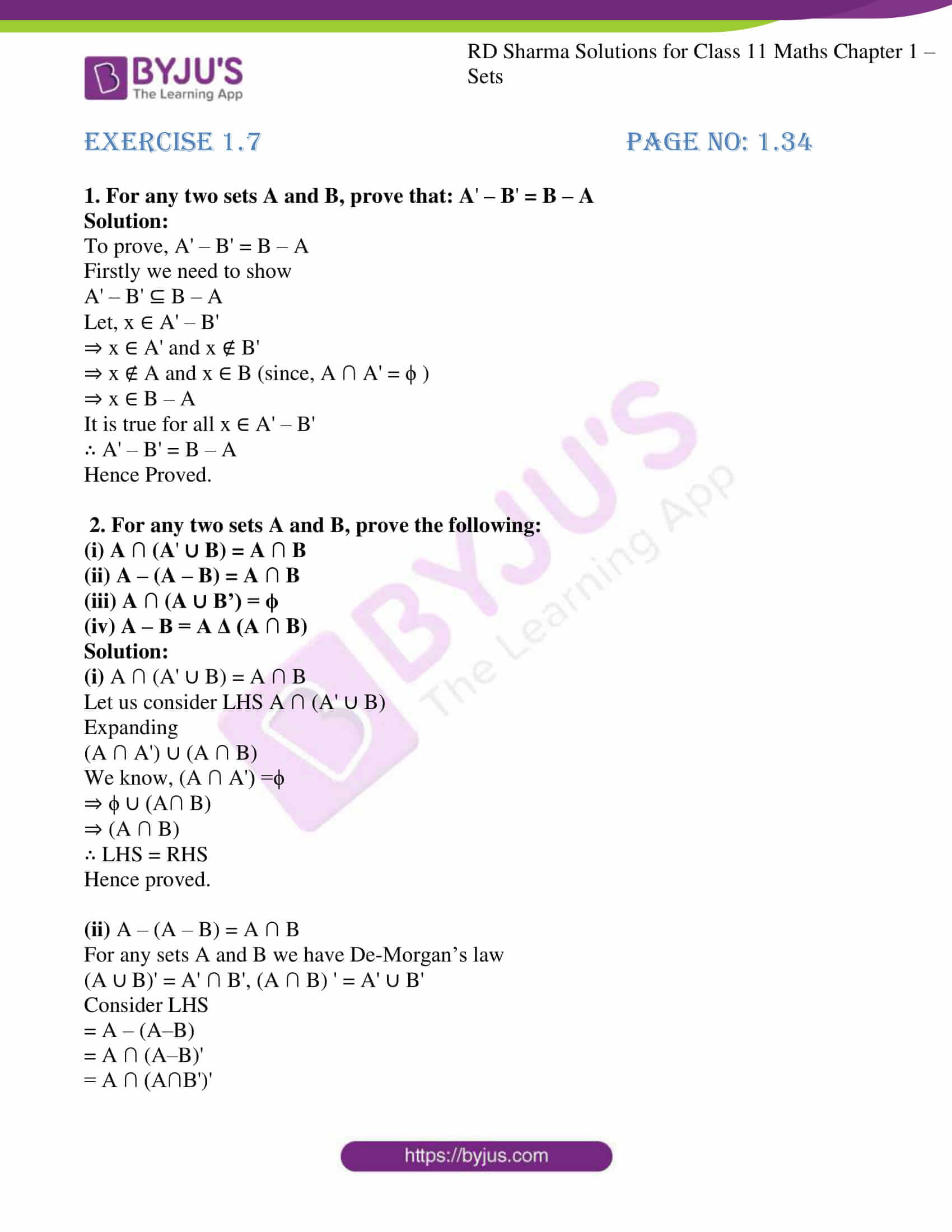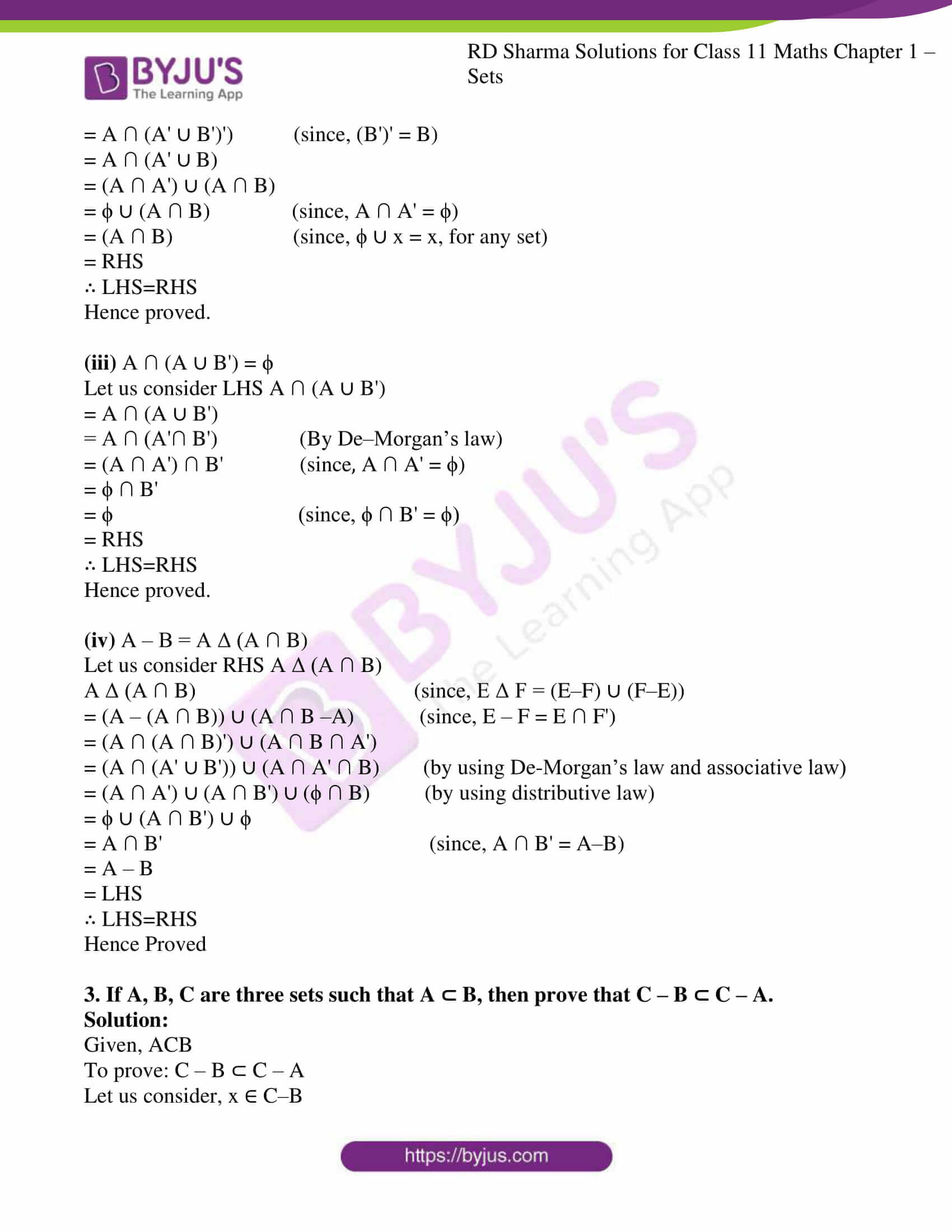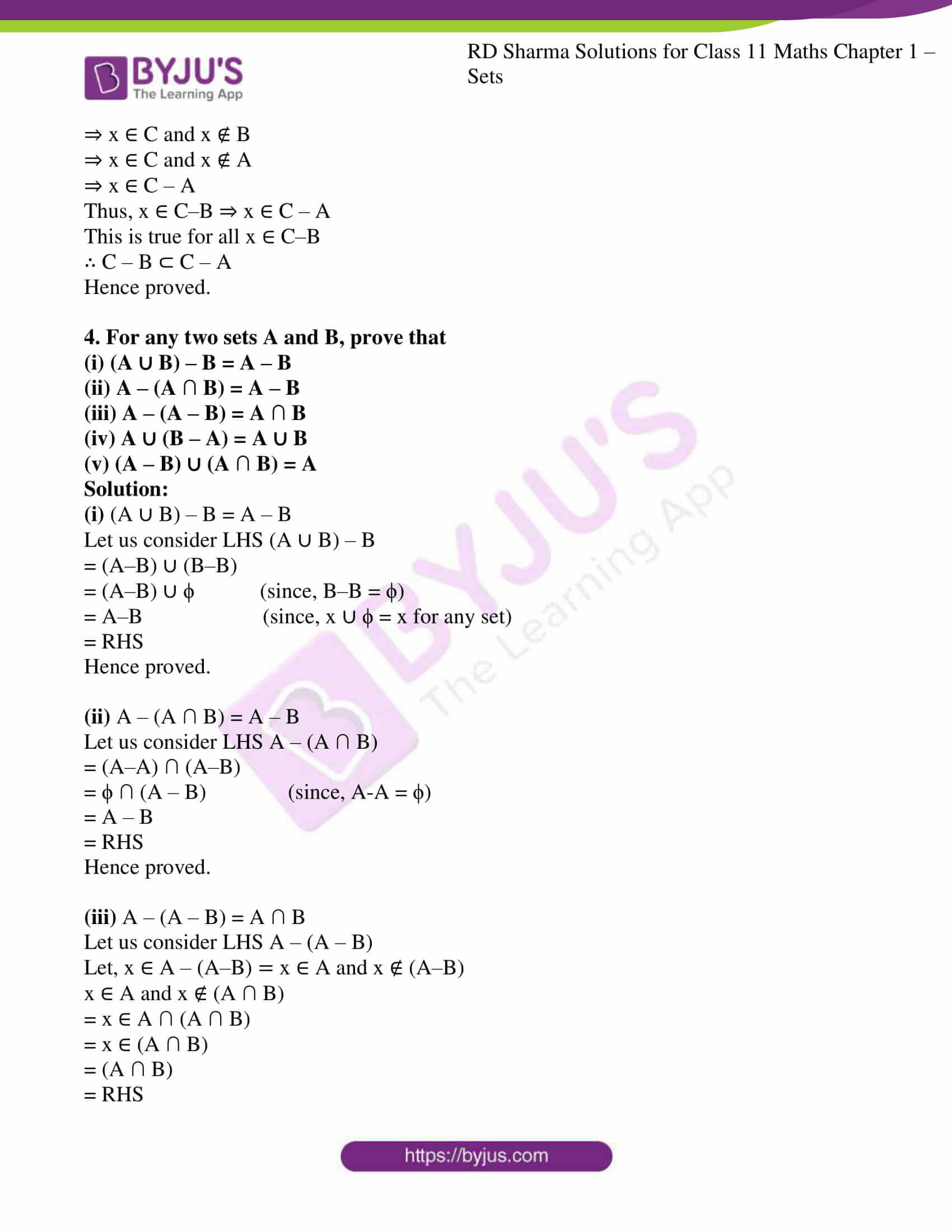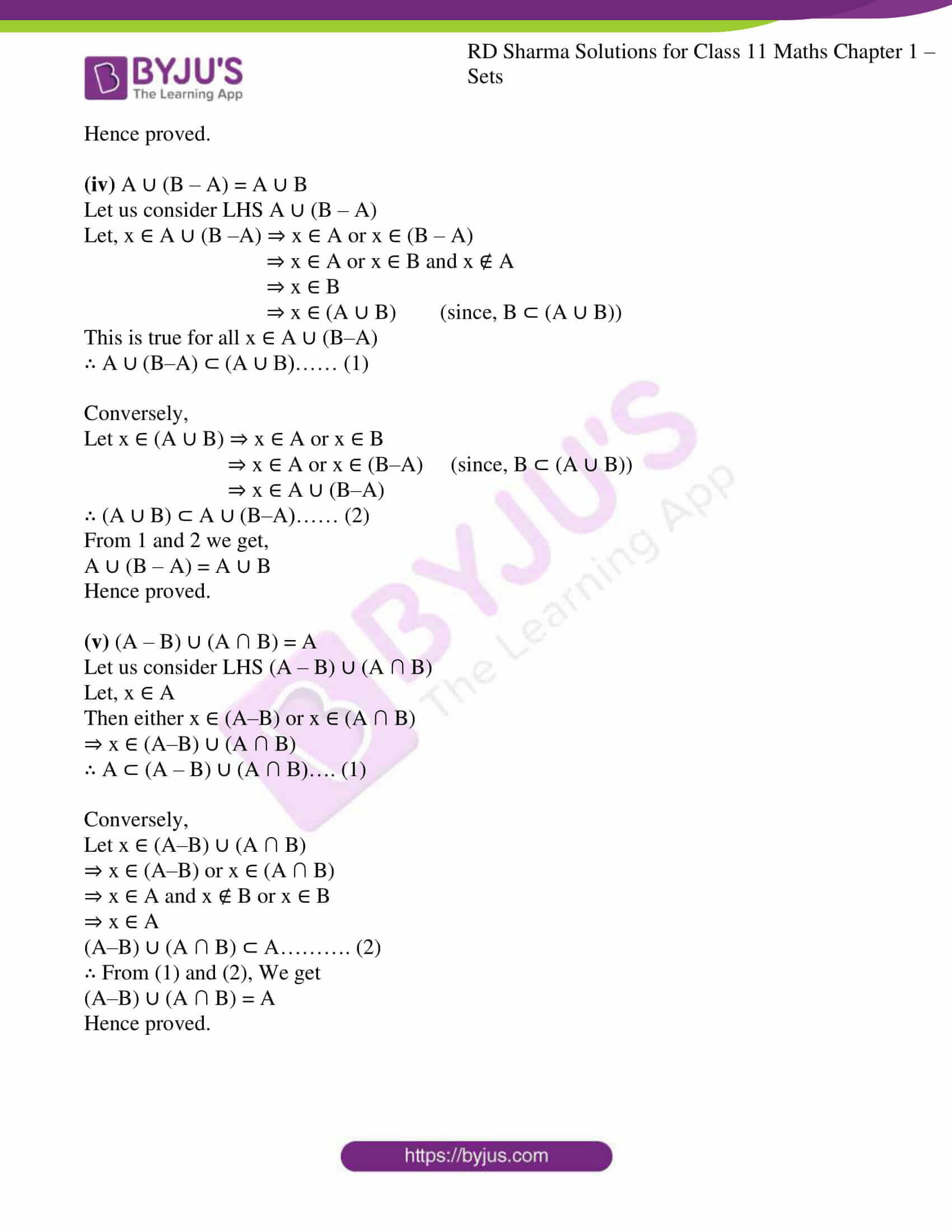# RD Sharma Solutions for Class 11 Chapter 1 - Sets Exercise 1.7

In Exercise 1.7, we shall see more results on operations on sets. Students who are finding difficulty in understanding the concepts can refer to RD Sharma Class 11 Solutions which are prepared by expert tutors at BYJU’S in a simple and understandable language. For further assistance, students can download the RD Sharma Class 11 solutions, which are available in the pdf format for better understanding and score well in their board exams.

## Download the Pdf of RD Sharma Solutions for Class 11 Maths Exercise 1.7 Chapter 1 – Sets### Access answers to RD Sharma Solutions for Class 11 Maths Exercise 1.7 Chapter 1 – Sets

1. For any two sets A and B, prove that: A – B = B – A

Solution:

To prove, A’ – B’ = B – A

Firstly we need to show

A’ – B’ ⊆ B – A

Let, x ∈ A’ – B’

⇒ x ∈ A’ and x ∉ B’

⇒ x ∉ A and x ∈ B (since, A ∩ A’ = ϕ )

⇒ x ∈ B – A

It is true for all x ∈ A’ – B’

∴ A’ – B’ = B – A

Hence Proved.

2. For any two sets A and B, prove the following:
(i) A ∩ (A
∪ B) = A ∩ B

(ii) A – (A – B) = A ∩ B

(iii) A ∩ (A ∪ B’) = ϕ

(iv) A – B = A Δ (A ∩ B)

Solution:

(i) A ∩ (A’ ∪ B) = A ∩ B

Let us consider LHS A ∩ (A’ ∪ B)

Expanding

(A ∩ A’) ∪ (A ∩ B)

We know, (A ∩ A’) =ϕ

⇒ ϕ ∪ (A∩ B)

⇒ (A ∩ B)

∴ LHS = RHS

Hence proved.

(ii) A – (A – B) = A ∩ B

For any sets A and B we have De-Morgan’s law

(A ∪ B)’ = A’ ∩ B’, (A ∩ B) ‘ = A’ ∪ B’

Consider LHS

= A – (A–B)

= A ∩ (A–B)’

= A ∩ (A∩B’)’

= A ∩ (A’ ∪ B’)’) (since, (B’)’ = B)

= A ∩ (A’ ∪ B)

= (A ∩ A’) ∪ (A ∩ B)

= ϕ ∪ (A ∩ B) (since, A ∩ A’ = ϕ)

= (A ∩ B) (since, ϕ ∪ x = x, for any set)

= RHS

∴ LHS=RHS

Hence proved.

(iii) A ∩ (A ∪ B’) = ϕ

Let us consider LHS A ∩ (A ∪ B’)

= A ∩ (A ∪ B’)

= A ∩ (A’∩ B’) (By De–Morgan’s law)

= (A ∩ A’) ∩ B’ (since, A ∩ A’ = ϕ)

= ϕ ∩ B’

= ϕ (since, ϕ ∩ B’ = ϕ)

= RHS

∴ LHS=RHS

Hence proved.

(iv) A – B = A Δ (A ∩ B)

Let us consider RHS A Δ (A ∩ B)

A Δ (A ∩ B) (since, E Δ F = (E–F) ∪ (F–E))

= (A – (A ∩ B)) ∪ (A ∩ B –A) (since, E – F = E ∩ F’)

= (A ∩ (A ∩ B)’) ∪ (A ∩ B ∩ A’)

= (A ∩ (A’ ∪ B’)) ∪ (A ∩ A’ ∩ B) (by using De-Morgan’s law and associative law)

= (A ∩ A’) ∪ (A ∩ B’) ∪ (ϕ ∩ B) (by using distributive law)

= ϕ ∪ (A ∩ B’) ∪ ϕ

= A ∩ B’ (since, A ∩ B’ = A–B)

= A – B

= LHS

∴ LHS=RHS

Hence Proved

3. If A, B, C are three sets such that A ⊂ B, then prove that C – B ⊂ C – A.

Solution:

Given, ACB

To prove: C – B ⊂ C – A

Let us consider, x ∈ C–B

⇒ x ∈ C and x ∉ B

⇒ x ∈ C and x ∉ A

⇒ x ∈ C – A

Thus, x ∈ C–B ⇒ x ∈ C – A

This is true for all x ∈ C–B

∴ C – B ⊂ C – A

Hence proved.

4. For any two sets A and B, prove that
(i) (A ∪ B) – B = A – B

(ii) A – (A ∩ B) = A – B

(iii) A – (A – B) = A ∩ B

(iv) A ∪ (B – A) = A ∪ B

(v) (A – B) ∪ (A ∩ B) = A

Solution:

(i) (A ∪ B) – B = A – B

Let us consider LHS (A ∪ B) – B

= (A–B) ∪ (B–B)

= (A–B) ∪ ϕ (since, B–B = ϕ)

= A–B (since, x ∪ ϕ = x for any set)

= RHS

Hence proved.

(ii) A – (A ∩ B) = A – B

Let us consider LHS A – (A ∩ B)

= (A–A) ∩ (A–B)

= ϕ ∩ (A – B) (since, A-A = ϕ)

= A – B

= RHS

Hence proved.

(iii) A – (A – B) = A ∩ B

Let us consider LHS A – (A – B)

Let, x ∈ A – (A–B) = x ∈ A and x ∉ (A–B)

x ∈ A and x ∉ (A ∩ B)

= x ∈ A ∩ (A ∩ B)

= x ∈ (A ∩ B)

= (A ∩ B)

= RHS

Hence proved.

(iv) A ∪ (B – A) = A ∪ B

Let us consider LHS A ∪ (B – A)

Let, x ∈ A ∪ (B –A) ⇒ x ∈ A or x ∈ (B – A)

⇒ x ∈ A or x ∈ B and x ∉ A

⇒ x ∈ B

⇒ x ∈ (A ∪ B) (since, B ⊂ (A ∪ B))

This is true for all x ∈ A ∪ (B–A)

∴ A ∪ (B–A) ⊂ (A ∪ B)…… (1)

Conversely,

Let x ∈ (A ∪ B) ⇒ x ∈ A or x ∈ B

⇒ x ∈ A or x ∈ (B–A) (since, B ⊂ (A ∪ B))

⇒ x ∈ A ∪ (B–A)

∴ (A ∪ B) ⊂ A ∪ (B–A)…… (2)

From 1 and 2 we get,

A ∪ (B – A) = A ∪ B

Hence proved.

(v) (A – B) ∪ (A ∩ B) = A

Let us consider LHS (A – B) ∪ (A ∩ B)

Let, x ∈ A

Then either x ∈ (A–B) or x ∈ (A ∩ B)

⇒ x ∈ (A–B) ∪ (A ∩ B)

∴ A ⊂ (A – B) ∪ (A ∩ B)…. (1)

Conversely,

Let x ∈ (A–B) ∪ (A ∩ B)

⇒ x ∈ (A–B) or x ∈ (A ∩ B)

⇒ x ∈ A and x ∉ B or x ∈ B

⇒ x ∈ A

(A–B) ∪ (A ∩ B) ⊂ A………. (2)

∴ From (1) and (2), We get

(A–B) ∪ (A ∩ B) = A

Hence proved.